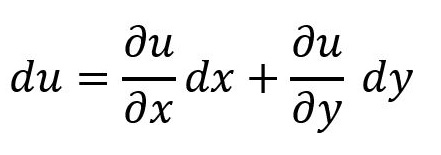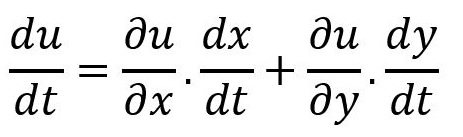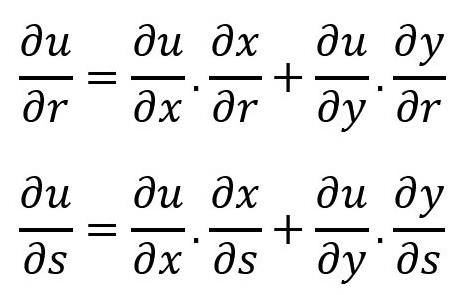## Differentiation of composite functions

If u=f(x_1 y)then total differential equation of ‘u’ is given by## Type 1 : Total derivative rule

If u=f(x,y) where x=x(t) and y=y(t),then## Type 2 : Total derivative Chain rule

If u=f(x,y) where x=x(r,s) and y=y(r,s),then
u is a function of (x,y),x and y are dependant on (r,s),hence
u becomes a function depndant on (r,s)